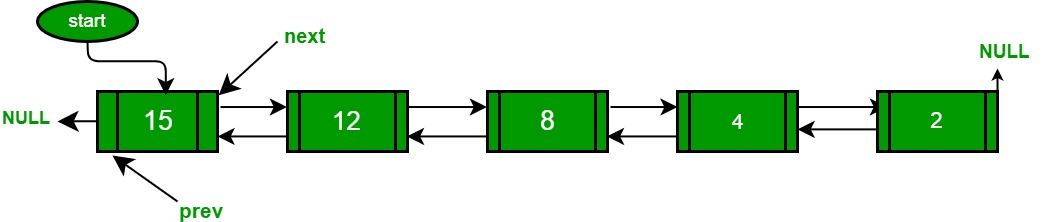GeeksforGeeks App
Open AppBrowser
Continue

# C++ Program For QuickSort On Doubly Linked List

Following is a typical recursive implementation of QuickSort for arrays. The implementation uses last element as pivot.

## C++

 `/* A typical recursive implementation of Quicksort for array*/` `/* This function takes last element as pivot, places the pivot element at its``   ``correct position in sorted array, and places all smaller (smaller than``   ``pivot) to left of pivot and all greater elements to right of pivot */``int` `partition (``int` `arr[], ``int` `l, ``int` `h)``{``    ``int` `x = arr[h];``    ``int` `i = (l - 1);`  `    ``for` `(``int` `j = l; j <= h- 1; j++)``    ``{``        ``if` `(arr[j] <= x)``        ``{``            ``i++;``            ``swap (&arr[i], &arr[j]);``        ``}``    ``}``    ``swap (&arr[i + 1], &arr[h]);``    ``return` `(i + 1);``}` `/* A[] --> Array to be sorted, l  --> Starting index, h  --> Ending index */``void` `quickSort(``int` `A[], ``int` `l, ``int` `h)``{``    ``if` `(l < h)``    ``{       ``        ``int` `p = partition(A, l, h); ``/* Partitioning index */``        ``quickSort(A, l, p - 1); ``        ``quickSort(A, p + 1, h);``    ``}``}`

Can we use the same algorithm for Linked List?
Following is C++ implementation for the doubly linked list. The idea is simple, we first find out pointer to the last node. Once we have a pointer to the last node, we can recursively sort the linked list using pointers to first and last nodes of a linked list, similar to the above recursive function where we pass indexes of first and last array elements. The partition function for a linked list is also similar to partition for arrays. Instead of returning index of the pivot element, it returns a pointer to the pivot element. In the following implementation, quickSort() is just a wrapper function, the main recursive function is _quickSort() which is similar to quickSort() for array implementation.## C++

 `// A C++ program to sort a linked list using Quicksort``#include ``using` `namespace` `std;` `/* a node of the doubly linked list */``class` `Node``{``    ``public``:``    ``int` `data;``    ``Node *next;``    ``Node *prev;``};` `/* A utility function to swap two elements */``void` `swap ( ``int``* a, ``int``* b )``{ ``int` `t = *a; *a = *b; *b = t; }` `// A utility function to find``// last node of linked list``Node *lastNode(Node *root)``{``    ``while` `(root && root->next)``        ``root = root->next;``    ``return` `root;``}` `/* Considers last element as pivot,``places the pivot element at its``correct position in sorted array,``and places all smaller (smaller than``pivot) to left of pivot and all greater``elements to right of pivot */``Node* partition(Node *l, Node *h)``{``    ``// set pivot as h element``    ``int` `x = h->data;` `    ``// similar to i = l-1 for array implementation``    ``Node *i = l->prev;` `    ``// Similar to "for (int j = l; j <= h- 1; j++)"``    ``for` `(Node *j = l; j != h; j = j->next)``    ``{``        ``if` `(j->data <= x)``        ``{``            ``// Similar to i++ for array``            ``i = (i == NULL)? l : i->next;` `            ``swap(&(i->data), &(j->data));``        ``}``    ``}``    ``i = (i == NULL)? l : i->next; ``// Similar to i++``    ``swap(&(i->data), &(h->data));``    ``return` `i;``}` `/* A recursive implementation``of quicksort for linked list */``void` `_quickSort(Node* l, Node *h)``{``    ``if` `(h != NULL && l != h && l != h->next)``    ``{``        ``Node *p = partition(l, h);``        ``_quickSort(l, p->prev);``        ``_quickSort(p->next, h);``    ``}``}` `// The main function to sort a linked list.``// It mainly calls _quickSort()``void` `quickSort(Node *head)``{``    ``// Find last node``    ``Node *h = lastNode(head);` `    ``// Call the recursive QuickSort``    ``_quickSort(head, h);``}` `// A utility function to print contents of arr``void` `printList(Node *head)``{``    ``while` `(head)``    ``{``        ``cout << head->data << ``" "``;``        ``head = head->next;``    ``}``    ``cout << endl;``}` `/* Function to insert a node at the``beginning of the Doubly Linked List */``void` `push(Node** head_ref, ``int` `new_data)``{``    ``Node* new_node = ``new` `Node; ``/* allocate node */``    ``new_node->data = new_data;` `    ``/* since we are adding at the``    ``beginning, prev is always NULL */``    ``new_node->prev = NULL;` `    ``/* link the old list off the new node */``    ``new_node->next = (*head_ref);` `    ``/* change prev of head node to new node */``    ``if` `((*head_ref) != NULL) (*head_ref)->prev = new_node ;` `    ``/* move the head to point to the new node */``    ``(*head_ref) = new_node;``}` `/* Driver code */``int` `main()``{``    ``Node *a = NULL;``    ``push(&a, 5);``    ``push(&a, 20);``    ``push(&a, 4);``    ``push(&a, 3);``    ``push(&a, 30);` `    ``cout << "Linked List before sorting``";``    ``printList(a);` `    ``quickSort(a);` `    ``cout << "Linked List after sorting``";``    ``printList(a);` `    ``return` `0;``}` `// This code is contributed by rathbhupendra`

Output :

```Linked List before sorting
30  3  4  20  5